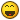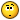# If a mathematical proof were to be dis proven by a counter example, what would that say about the foundations of Mathematics?

## Recommended Posts

Acme, I think your Easter hangover must still be with one of us.I think you will find the text you attributed to me was actually penned by SamBridge (post#20)

To continues with the geometry example I offered in post#12

Having defined a straight line in definition#4, Euclid went on to define a triangle in definition#19 as a trilateral rectilineal figure, contained within three straight lines.

The theorem (he called them postulates) he developed from his axioms and definitions was that the sum of the angles adds up to two right angles.

Now Euclid diod not know about spherical triangles, so if I offered a spherical triangle as a counterexample, disproving Euclid it is a good example of what ajb was talking about.

Eulcid remains valid, with the terms of the definitions, but spherical triangles failure to observe the two rightangles theorem demonstrates that this theorem should not be applied to non rectilineal figures.

However, if the theorem supported by a proof working off of he axioms of mathematics is disproved by a counterexample it would mean the axioms used are I'll founded, as others have stated.

So far as I can tell everyone is trying to tell you that the above is not so.

Axioms are stated as true statements, without proof and have some independence from each other.

Theorems are statements developed from these axioms in such as manner as to be true so long as the axioms are true.

This process of development is called proof.

A counterexample or other disproof would be of a theorem, not an axiom. You cannot disprove something you start out taking to be true, without proof.

Thus a disproof would be against your development process, not the axioms.

Of course you could one day find that your axiom has let you down and is indeed not true, which is why so much effort goes into testing them.

But it is also possible that you could find conditions/definitions outside the original ones. This is the situation ajb and I are trying to explore here.

Does this make sense?

Edited by studiot
##### Share on other sites

If the original proof was correct (logically following from the axioms) and the existence of a counterexample could be proven from the same axioms (again, assuming a correct proof) then the axiom system would be inconsistent.

Would this be a bad thing for math? Not really. The Zermelo-Fraenkel axioms of set theory are less than 100 years old (dating from 1922), yet mathematicians got along fine without them since ancient times. If ZF turns out to be inconsistent, foundationalists will just get busy fixing up new foundations. In fact this process is well underway. Serious people are already developing a brand new foundation for math not based on set theory

http://en.wikipedia.org/wiki/Homotopy_type_theory

But suppose set theory turns out to be inconsistent and no new foundations are available yet? It still won't trouble most working mathematicians. They'll still do their work in analysis, algebra, geometry, number theory, etc., just as they did before ZF.

After all, set theory may be inconsistent, but 5 is still a prime number. So people who study prime numbers won't be troubled in the least.

Edited by Someguy1
##### Share on other sites

Acme, I think your Easter hangover must still be with one of us.I think you will find the text you attributed to me was actually penned by SamBridge (post#20)

snip...But can you prove that? I fixed it. While I ground the wrong axe-on the quote, I was chopping the right would. I think I'll start that drinking now.

[Acme]That not even wrong statement does not apply here because there is no fallacy within the question. The idea of a proof is that it gives a foundation stating that something is always true and most likely will remain that way with current axioms of mathematics. However, if the theorem supported by a proof working off of he axioms of mathematics is disproved by a counterexample it would mean the axioms used are I'll founded, as others have stated.

The phrase not even wrong describes any argument that purports to be scientific but fails at some fundamental level, usually in that it contains a terminal logical fallacy or it cannot be falsified by experiment (i.e. tested with the possibility of being rejected), or cannot be used to make predictions about the natural world. ...

Your title fails at the fundamental level of definition. A mathematical proof by definition cannot have a counter example. Nothing of what you have said puts the foundations of mathematics in peril. Please spare me further retorts and call it a wash.

##### Share on other sites

Your title fails at the fundamental level of definition. A mathematical proof by definition cannot have a counter example. Nothing of what you have said puts the foundations of mathematics in peril. Please spare me further retorts and call it a wash.

I apologize for saying this, but it seems like you are trying to act like a jerk.In mathematics, a proof is a deductive argument for a mathematical statement. In the argument, other previously established statements, such as theorems, can be used. In principle, a proof can be traced back to self-evident or assumed statements, known as axioms.

I think this rests my case.

##### Share on other sites

> Your title fails at the fundamental level of definition. A mathematical proof by definition cannot have a counter example.

A mathematical proof most definitely can have a counterexample. Any inconsistent set of axioms will have lots of provable statements of the form "P and not-P". That's because an inconsistent system can prove anything. In fact the only way we can possibly ever hope to have a complete set of axioms for math is to start with an inconsistent set of axioms. That's Godel's incompleteness theorem. It says that the axioms of set theory are complete if and only if they're inconsistent.

Note that it doesn't say which of these two cases is true! Everyone assumes that set theory is incomplete. But it's possible that it's complete, but inconsistent.

To explain this in a bit more detail, what is a proof? A proof is a set of statements, each one following logically from the axioms and from previous statements. It says nothing about whether the axioms are self-contradictory. If they are, then you'll be able to prove P and not-P for any proposition P that you care to choose.

As far as whether the foundations of math are in peril, they most certainly are. As I said earlier, ZF is less than 100 years old. It's highly historically contingent. People did math for thousands of years without it.

Set theory as the foundation of math is already under genuine attack from a variety of directions. Computability and complexity theory, Category theory, and Homotopy Type Theory are already three promising avenues of research into alternate foundations.

Here is a thought question for you. Given any axiom system, a theorem has a proof. That's by definition of proof, which is "a statement that can be logically derived from the axioms." Therefore (once we fix our formal language) any theorem has a shortest proof.

Now, suppose that there is a contradiction in the set theory.

Therefore, some contradiction has a proof in ZF. Now: What happens if there is some provable contradiction in ZF; but the length of the shortest proof of that contradiction is larger than the number of quarks in the universe?

In that case set theory would be known to be inconsistent; but it wouldn't matter the slightest bit to anyone; because you can be certain that any proof that you can possibly write down, is not a contradiction.

Inconsistent axiom systems are not meaningless or trivial. They turn out to be interesting!

Edited by Someguy1
##### Share on other sites

P and NOTP can only take you so far.

Every designer of electronic logic circuits knows (or should know) that although logic circuits are based on the same propositional calculus as P & NOTP, there is a third option, known as tristate.

##### Share on other sites

I apologize for saying this, but it seems like you [Acme] are trying to act like a jerk.No apology necessary. I was simply employing some hyperbole for rhetorical/stylistic purpose. I only mean to sharpen your wits.In mathematics, a proof is a deductive argument for a mathematical statement. In the argument, other previously established statements, such as theorems, can be used. In principle, a proof can be traced back to self-evident or assumed statements, known as axioms.

I think this rests my case.

And I thought this arrested mine.

I mean the word proof not in the sense of lawyers, who set two half proofs equal to a whole one, but in the sense of a mathematician, where half proof = 0, and it is demanded for proof that every doubt becomes impossible.

## Create an account

Register a new account